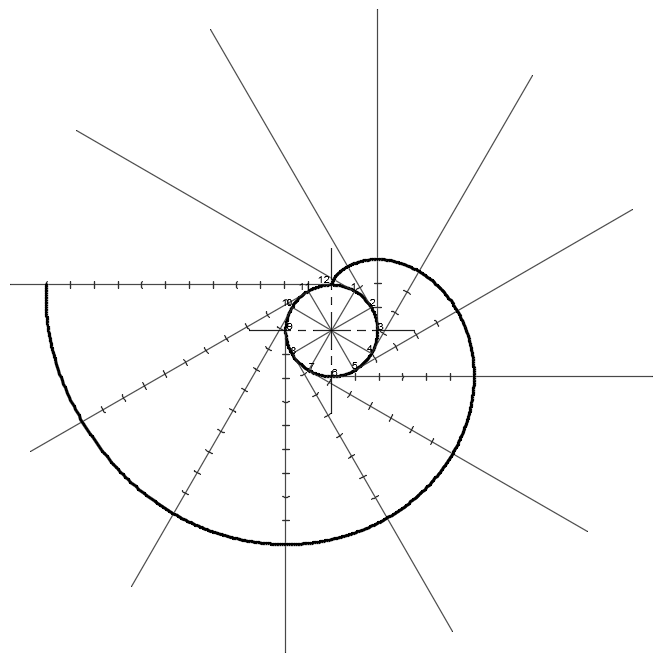Geometry > The Involute of a circle

Learning outcome:
• I can trace and name the locus of a string end as it unwinds from a plane geometric figure i.e. involute.

By the end of the lesson I will be able to:

• Draw one revolution of an involute
• Draw a part revolution of an involute (or a combination of)
• Design my own shapes using the involutes

Before studying this lesson I need to make sure that I know how to:

• Draw a center line
• Draw a neat freehand curve
• Divide the circle into 12 equal parts
• Divide a line into 12 equal parts
• Calculate the circumference of a circle (2pr)The Involute of a circle

The involute of a circle is the locus (path) traced by the end of a string as it is unwound from around the circle. To draw the involute using graphical means, we have to divide the circle into a number of equal parts (usually 12) and add tangents to each point. These tangents represent the string that is being unwound, at various stages. Mark off the lengths of the string at each point by adding 1/12th of the circumference each time the string unwinds 1/12th of a revolution. The involute is to be drawn freehand by going through these points.

To see an example of this you can visit:

Note that the line forming the involute is the green one as it is the one that lies on the end of the string.